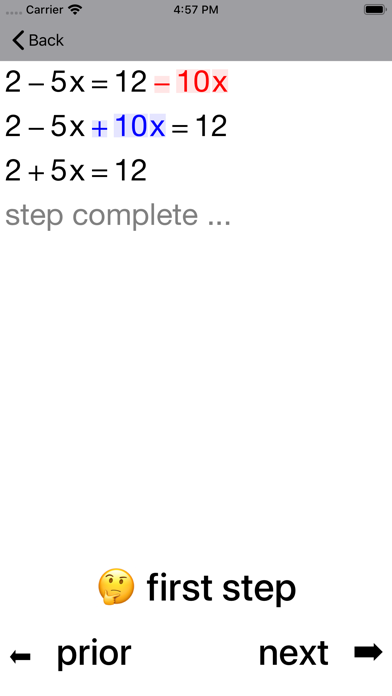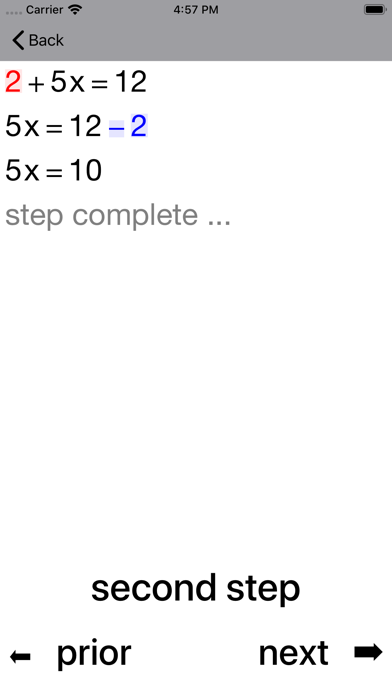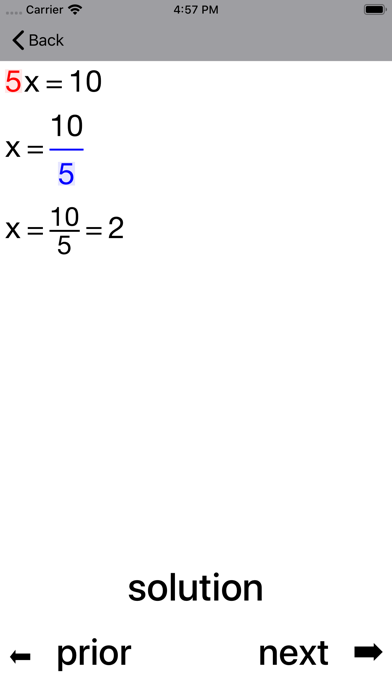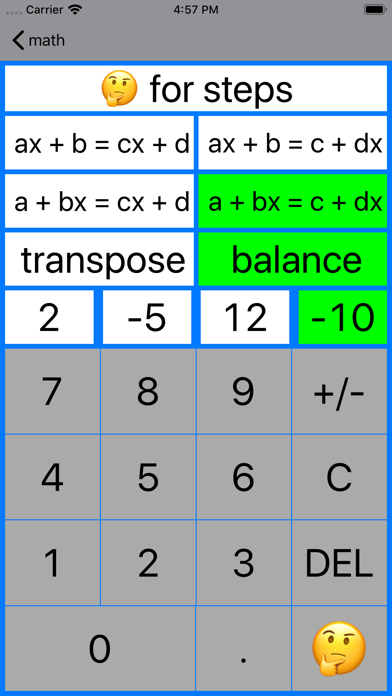## 20/20 Linear Equations - Lumos Educational App Store11
Price -\$0.99
\$0.99

#### DESCRIPTION:

A power learning aid combining Coaching Calculators, Practice and Guide to help students master linear equations. Coaching Calculators focus on one-step, two-step and multi-step equations such as x + 6 = 9, x/2 − 5 = 6 and 5x + 2 = 3x + 8 respectively. One-step x − 2 = 3, 5 + x = 4, 3x = -12, x/7 = 2 Two-step 3x + 8 = 20, x/9 + 2 = 5 Multi-step 4 + 9x = 2x + 18 The Practice unit focuses on equations with parentheses and fractions along with one-step, two-step and multi-step equations. Equations with parentheses and fractions 10 − 3(2 − 3x) = 2x + 9 (1/2)(2x − 5) = 3x + 3/

#### OVERVIEW:

20/20 Linear Equations is a free educational mobile app By Walter Kissach.It helps students practice the following standards .

This page not only allows students and teachers download 20/20 Linear Equations but also find engaging Sample Questions, Videos, Pins, Worksheets, Books related to the following topics.

Developer: Walter Kissach

Developer URL: NA

Software Version: 6.0

Category: Education

Release Date: 2009-05-08T21:28:47Z#### Are you the Developer?EdSearch WebSearch